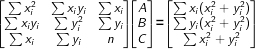# Least-Squares Circle Calculator

You can use this least-squares circle calculator to identify the circle that fits the provided points in the plane most effectively from the least-squares perspective.

You can use this calculator in two simple steps:

1. Input the X and Y data pairs in the fields provided.
2. Click on the "Calculate" button, and the calculator will determine the coordinates of the radius and center of the circle.
Least-Squares Circle Calculator

## Understanding the Best Fit Circle

In a situation in which you have the data points x, y that are distributed in a ring-shape on an x-y plane, the least-squares regression can be used to determine the equation of a circle that will best fit with the available data points; i.e., the following regression will help you to calculate the k, m, and r values of the curve:

(x − k)2 + (y − m)2 = r2

When you use the least-squares, you determine the "best fit" by minimizing the equation as follows:

F(k, m, r) = ∑[(xi − k)2 + (yi − m)2 − r2]2

The equation of the circle is linearized by the model ∂F/∂k = 0, ∂F/∂m = 0, and ∂F/∂r = 0. As such, we have the following:

(x − k)2 + (y − m)2 = r2

x2 − 2kx + k2 + y2 − 2my + m2 = r2

x2 + y2 = 2kx + 2my + r2 − k2 − m2

x2 + y2 = Ax + By + C

This results in a linear equation with the coefficients A, B, and C undetermined. As such, you can use the matrices to solve the least-squares problem. Once you have determined A, B, and C, it is possible to work backward to compute k, m, and r.

## Finding A, B, and C with Matrices

The circle regression model is supported by the following matrix equation:where n is the number of data points (xi, yi).

In a situation in which the 3-by-3 matrix outlined on the left is invertible, the A, B, and C values are unique, and this determines the best fit circle.

You can use the A, B, and C values to subsequently determine k, m, and r:

k = A/2

m = B/2

r = (√4C + A² + B²)/2

Example:

Let's say we want to identify the equation of the circle that best fits the following four data points: (0, 1), (2, 5), (1, 6), and (7, 6).

The use of the matrix equation or the least squares circle calculator results in the following:

(x − 4.2408)2 + (y − 2.4630)2 = 4.32202.

You may also be interested in our Quadratic Regression Calculator or Linear Regression Calculator